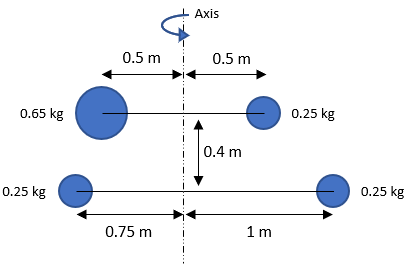## Mass Moment of Inertia

For the point mass figure below, what is the mass moment of inertia?Hint
For point masses, the mass moment of inertia is:
$$I=\sum m_ir_i^2$$$where $$m$$ is the mass, and $$r$$ is the perpendicular distance to the rotation axis. Hint 2 Ignore the 0.4 m distance. That dimension is there to throw you off. For point masses, the mass moment of inertia is: $$I=\sum m_ir_i^2$$$
where $$m$$ is the mass, and $$r$$ is the perpendicular distance to the rotation axis.
The figure has four point masses:
$$I=m_1r_{1}^{2}+m_2r_{2}^{2}+m_3r_{3}^{2}+m_4r_{4}^{2}$$$Remember, $$r$$ is the perpendicular distance the mass is from the rotation axis, not parallel distance, meaning the 0.4 m dimension is there for confusion and can be ignored. Starting from the bottom right point mass and moving clockwise: $$I=(0.25kg)(1m)^{2}+(0.25kg)(0.75m)^{2}+(0.65kg)(0.5m)^{2}+(0.25kg)(0.5m)^{2}$$$
$$=(0.25kg)(1m^2)+(0.25kg)(0.5625m^2)+(0.65kg)(0.25m^2)+(0.25kg)(0.25m^2)$$$$$=0.25kg\cdot m^2+0.140625kg\cdot m^2+0.1625kg\cdot m^2+0.0625kg\cdot m^2$$$
$$I=0.616\:kg\cdot m^2$$$$$0.616\:kg\cdot m^2$$$